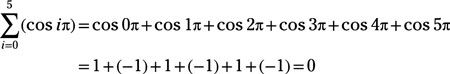##### Pre-Calculus: 1001 Practice Problems For Dummies (+ Free Online Practice)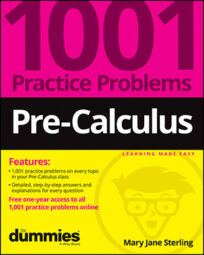A series is the sum of a list of numbers, such as 1 + 2 + 4 + 8. Many times, you can find a formula to help you add up the numbers in a series. Formulas are especially helpful when you have a lot of numbers to add or if they’re fractions or alternating negative and positive.

You can classify many series by type, which helps you determine particular terms or sums. Recognize the type, and you’re pretty much home free when doing your calculations.

You’ll work on series in the following ways:

• Becoming familiar with summation notation

• Computing the sum of terms in arithmetic and geometric series

• Generating terms by using the binomial theorem

Don’t let common mistakes trip you up; keep in mind that when working with series, some challenges will include

• Recognizing when a series is arithmetic or geometric

• Using the infinite series sum only when the ratio of consecutive terms has an absolute value less than 1

## Practice problems

1. Find the sum of the arithmetic series: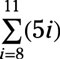You find the sum of an arithmetic series with the formula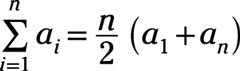where you’re averaging the first and last terms and multiplying by n, the number of terms.

In this series, you’re actually going from the eighth to the eleventh term, which means you’re adding four terms. Using the formula, n = 4, the eighth term is 40, and the eleventh term is 55: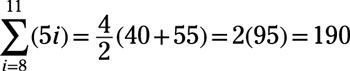2. Find the sum of the series.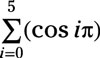Write out the six terms, compute the value of the trig function, and then add the terms: